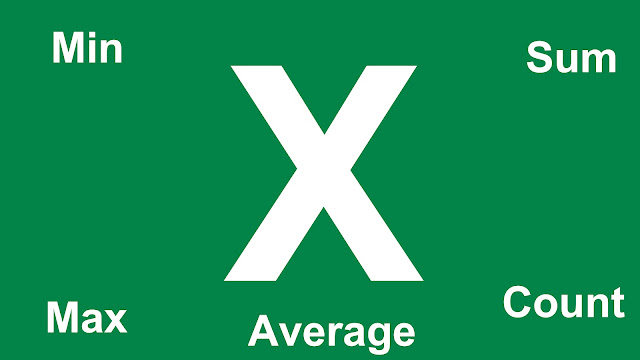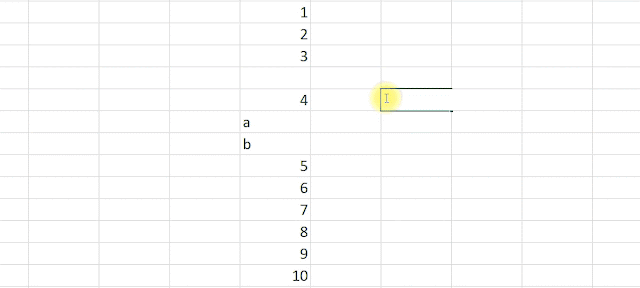This Domain is available for sale contact pakdevelopers.com.pk@gmail.com

# Microsoft Excel Some Basic Functions And Formulas For BeginnersIt is important to learn and know about excel formulas, if we take no interest in excel formulas. So, it is unusable for us, in this excel online article we will learn some basic formulas. Excel is a form of spreadsheet. If we entered the data in a tabular form so this is known to be spreadsheet. In Microsoft Excel, there is some formulas which are predefined called functions, means Microsoft programmed the formulas, so we only call it and enter the values in it in a predefined manner, if we not use this predefined manner, so we will face the error. It is very important to use the formulas in excel, to use the functions must type equals to sign (=) before starts the formula. Lets star the some basic functions.

Sum Formula:

This function of sum will sum all values that will select.

Example:

=sum(number1, number2,.....) ; general format

=sum(A1:A10) ; column wise format

=sum(a1:z1); row wise format

Average Formula
This function of average, will calculate the average value from the given values. This function in excel sum of all the values then divide by the total number number of values.

Example:
=average(value1, value2,...)

Count Formula:
This function counts all the numeric values (only numbers) in a row or column.

Example:
=count(value1, value2,...)Microsoft excel count function with alphanumeric and empty values

Counta Formula:
This function counts all the alphabetic and numeric values, which means alphanumeric values in a row or column.

Example:
=counta(value1, value2,...)

Countblank Formula:
This function counts all the empty cells between or after or before the values in a range.

Example:
=countblank(value1, value2,...)

Min Formula:
This function find the lowest value in a range.

Example:
=min(value1, value2,...)

Max Formula:
This function find the largest value in a range.

Example:
=max(value1, value2,...)